# Workflow to perform Global Sensitivity Analysis with the PAWN method¶

### (Department of Civil Engineering, University of Bristol, UK)¶

This document provides:

• a brief introduction to Global Sensitivity Analysis (GSA);
• a workflow to understand how the PAWN GSA method works, to guide in its application using the SAFE (Sensitivity Analysis For Everybody) toolbox (References 1-2), and to interpret the results.

In this example we apply the PAWN method (Reference 3) to the Ishigami-Homma test function. The steps are based on the workflow_pawn_ishigami_homma available in the SAFE toolbox.

# PART I: Introduction¶

## What is Global Sensitivity Analysis and why shall we use it?¶

Global Sensitivity Analysis is a set of mathematical techniques which investigate how the uncertainty in the model inputs influences the variability of the model outputs (References 4-5).

The benefits of applying GSA are:

• Gain a better understanding of the model: Evaluate the model behaviour beyond default set-up.

• Get a "sanity check” of the model: Identify unexpected model responses so to guide the model debugging and the definition of its scope of validity.

• Identify priorities for uncertainty reduction: Identify the important inputs on which to focus acquisition of new data, computationally intensive calibration, etc.

• Make more transparent and robust decisions: Understand how assumptions about uncertain inputs reflect on the modelling outcome and thus on model-informed decisions.

## How Global Sensitivity Analysis works¶

The figure below summarises the main steps to apply GSA to a generic cat model (References 5-6).

An 'input factor' is any element that can be changed before running the model. In general, input factors could be the model’s parameters, forcing input data, but also the very equations implemented in the model or other set-up choices (for instance, the spatial resolution) needed for the model execution on a computer.

An 'output' is any variable that is obtained after the model execution.

The key steps of GSA are summarised in the figure below.

In :
import os
mydir = "C:/Users/valen/OneDrive - University of Bristol/proj_SAFEVAL/SAFEPy/SAFEpython_v0.0.0"
os.chdir(mydir + "/jupyter")
from IPython.display import Image
Image(filename="how_GSA_works.png", width=800, height=800)

Out: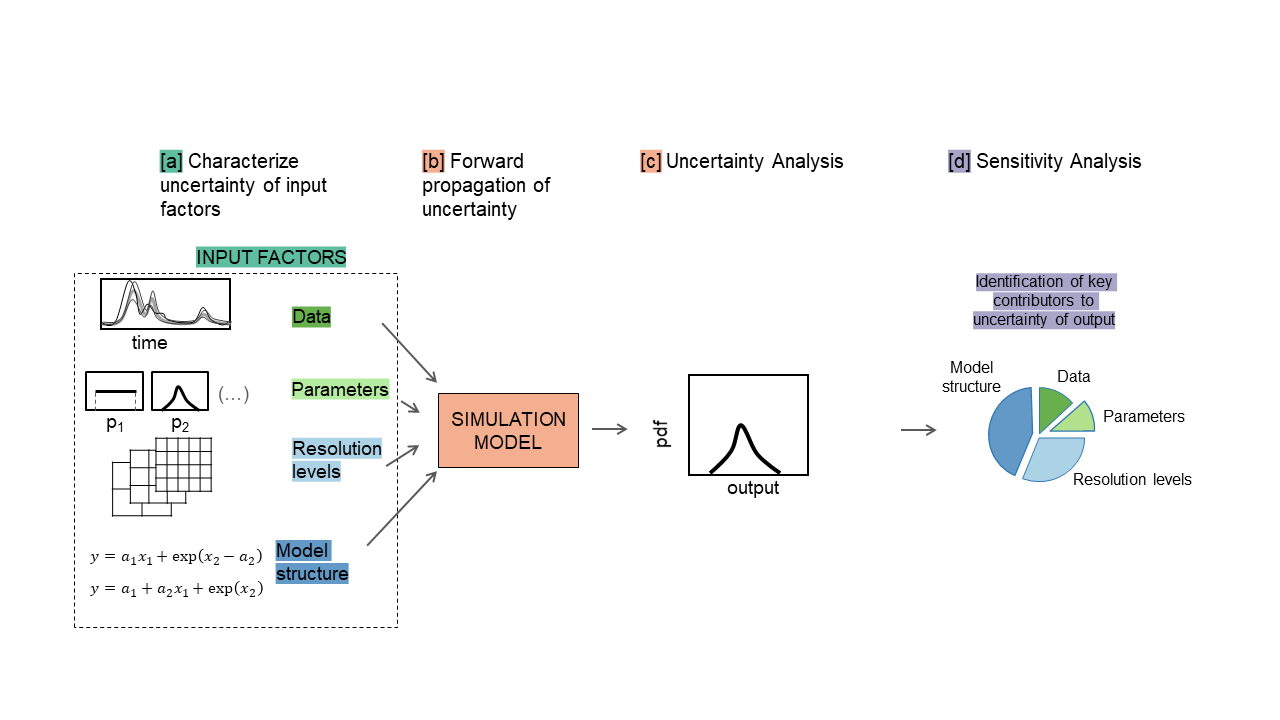a. The uncertainty in each input factor is characterised by a range of variability or a probability distribution, and a number of input factor combinations are sampled from such ranges/distributions.

b. The model is repeatedly run against each of the sampled combinations of input factors.

c. The output samples so obtained can be used to visually explore the output uncertainty, for instance through a probability distribution plot or a set of scatter plots.

d. Input and output samples are quantitatively analysed in order to obtain a set of sensitivity indices. The sensitivity indices measure the relative contribution of each input factor to the output uncertainty.

# PART II: Workflow application¶

### Step 1: Import python modules¶

In :
from __future__ import division, absolute_import, print_function

import numpy as np
import matplotlib as mpl
import matplotlib.pyplot as plt
import scipy.stats as st

os.chdir(mydir + "/SAFEpython")

# Import SAFE modules:
from SAFEpython import PAWN
import SAFEpython.plot_functions as pf # module to visualize the results
from SAFEpython.model_execution import model_execution # module to execute the model
from SAFEpython.sampling import AAT_sampling, AAT_sampling_extend # module to perform the input sampling
from SAFEpython.util import aggregate_boot  # function to aggregate the bootstrap results

from SAFEpython.ishigami_homma import ishigami_homma_function

os.chdir(mydir)


#### Test function: Ishigami-Homma¶

Before applying GSA, let's have a brief overview of the Ishigami-Homma function, which is a standard benchmark function in the Sensitivity Analysis literature, given that the output variance and the first and total-order sensitivity indices can be computed analytically (see for instance Eq. (4.34) in (Reference 7):

$$y = sinx_1 + asin^2x_2 + bx_3^4sinx_1$$

### Step 2: Setup the model¶

Define:

• the input factors whose influence is to be analysed with GSA,
• their range of variability (choice made by expert judgement, available data or previous studies),
• choice of their distributions.
In :
# Number of uncertain parameters subject to SA:
M = 3

# Parameter ranges:
xmin = -np.pi
xmax = np.pi
# Parameter distributions:
distr_fun = st.uniform # uniform distribution
# distr_fun = st.randint # for discrete distribution

# The shape parameters of the uniform distribution are the lower limit and the difference between lower and upper limits:
distr_par = [xmin, xmax - xmin]
# The shape parameters of the discrete uniform distribution are the lower limit and the upper limit+1:
# distr_par = [min, max+1]

# Define output:
fun_test = ishigami_homma_function


### Step 3: Sample inputs space¶

The number of model runs (N) typically increases proportionally to the number of input factors (M) and will depend on the GSA methods chosen as well.

As a rule of thumb, it may require more than 10 model runs per input factor (M) for the most frugal methods (e.g. Elementary Effect Test) and more than 1000 model runs per M for the more expensive methods (e.g. variance and density-based methods).

In :
samp_strat = 'lhs' # Latin Hypercube
N = 2000  #  Number of samples
X = AAT_sampling(samp_strat, M, distr_fun, distr_par, N)


### Step 4: Run the model¶

For each sampled input factors combination, we run the model and save the associated model output.

In :
Y = model_execution(fun_test, X)


## Apply the PAWN Global Sensitivity Analysis method¶

Let’s now apply Global Sensitivity Analysis, here the PAWN method.

Its main idea is that the influence of an input factor is proportional to the amount of change in the output distribution produced by fixing that input.
The sensitivity of $y$ to $x_{i}$ is measured by the difference between the unconditional CDF of $y$: $F_y( y )$, which is induced by varying all input factors simultaneously, and the conditional CDF that is obtained by varying all inputs but $x_{i}$: $F_{y|x_i}( y | x_i )$.

### Step 5: Check model behaviour by visualising input/output samples¶

In :
X_Labels=['$x_{1}$', '$x_{2}$', '$x_{3}$']

# Set figures sizes
plt.rcParams['figure.figsize'] = [15, 5]
pltfont = {'fontname': 'DejaVu Sans', 'fontsize': 15} # font

pf.scatter_plots(X, Y, Y_Label='y', X_Labels=X_Labels)
plt.show()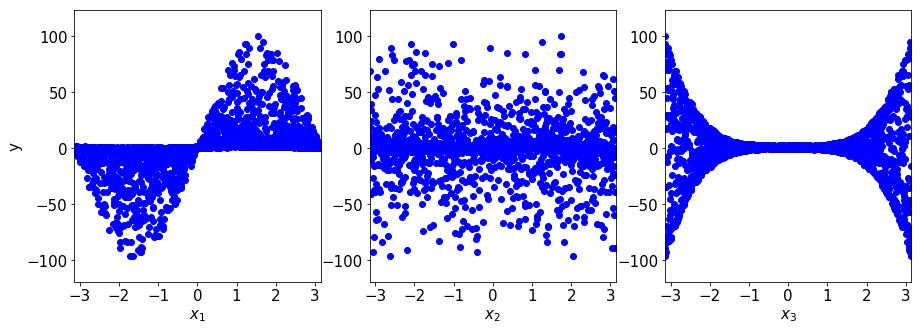Question:

From these scatter plots, which input factor(s) would you say is influential? Why?

Are there input factors that are non influential at all?

$x_{1}$ and $x_{3}$ are both influential, as their impact on $y$ varies along the horizontal axis, e.g. positive values of $x_{1}$ increases $y$, while negative ones decreases $y$, while for $x_{3}$ its extreme values increase the variability of $y$.

$x_{2}$ is not influential, as any value has the same impact on the output $y$.

### Split samples into n groups and re-plot scatterplots¶

(This step is needed only to produce the visualisation below, which aids the explaination of the PAWN method.

Let's split the samples into $n$ equiprobable intervals (i.e. with approximately the same number of data points in each interval).

Unconditional output sampled are in red, while conditional output samples are in grey.

In :
n = 10 # number of equiprobable conditioning intervals

YY, xc, NC, n_eff, Xk, XX = PAWN.pawn_split_sample(X, Y, n)

# Determine the sample size for the unconditional output bootsize:
bootsize = [int(np.min(i)) for i in NC] # numpy.ndarray(M,)
# bootsize is equal to the sample size of the conditional outputs NC, or
# its  minimum value across the conditioning intervals when the sample size
# varies across conditioning intervals as may happen when values of an
# input are repeated several times (more details on this in the Note in the
# help of the function).

# To reduce the computational time (the calculation of empirical CDF is
# costly), the unconditional CDF is computed only once for all inputs that
# have the same value of bootsize[i].
bootsize_unique = np.unique(bootsize)
N_compute = len(bootsize_unique) # number of unconditional CDFs that will be computed for each bootstrap resample

# Compute sensitivity indices with bootstrapping
for kk in range(N_compute):
# Bootstrap resampling (Extract an unconditional sample of size bootsize_unique[kk]
# by drawing data points from the full sample Y without replacement
idx_bootstrap = np.random.choice(np.arange(0, N, 1), size=(bootsize_unique[kk], ), replace='False')

colorscale = 'gray'

for i in range(M):

cmap = mpl.cm.get_cmap(colorscale, n_eff[i]+1) # return colormap,
# (n+1) so that the last line is not white
# Make sure that subsample centers are ordered:

plt.subplot(1, 3, i+1)
if ((i+1) % 3) == 1: # first column
plt.ylabel('y', **pltfont)
plt.xlabel(X_Labels[i], **pltfont)
plt.xlim((np.min(X[:, i]), np.max(X[:, i])))
plt.ylim((np.min(Y) - np.std(Y), np.max(Y) + np.std(Y)))
plt.xticks(**pltfont); plt.yticks(**pltfont)
for k in range(n_eff[i]):
plt.plot(XX[i][k][:,i], YY[i][k], 'o', markerfacecolor=cmap(k), markeredgecolor=cmap(k)) # plot conditional values

plt.plot(X[idx_bootstrap, i], Y[idx_bootstrap], 'o', markerfacecolor='red', alpha=0.2, markeredgecolor='red') # plot unconditional values
for j in range(np.shape(Xk)):
plt.axvline(x = Xk[i][j], color = 'gray', ls = '--', lw = 0.5) # plot vertical lines at the interval edges
plt.show()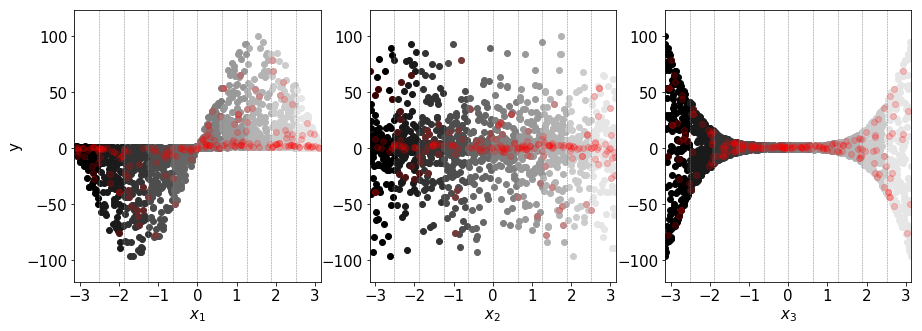### Step 6: Derive unconditional and conditional output distributions¶

Here we compute and plot unconditional and conditional output CDFs, where the conditional CDFs are obtained by fixing $x_{i}$ to one of the $n$ equiprobable intervals.

In :
# Compute and plot conditional and unconditional CDFs:
YF, FU, FC, xc = PAWN.pawn_plot_cdf(X, Y, n, cbar=True, n_col=3, labelinput=X_Labels)
plt.subplots_adjust(left=None, bottom=None, right=None, top=None, hspace=0, wspace=0.4)
plt.show()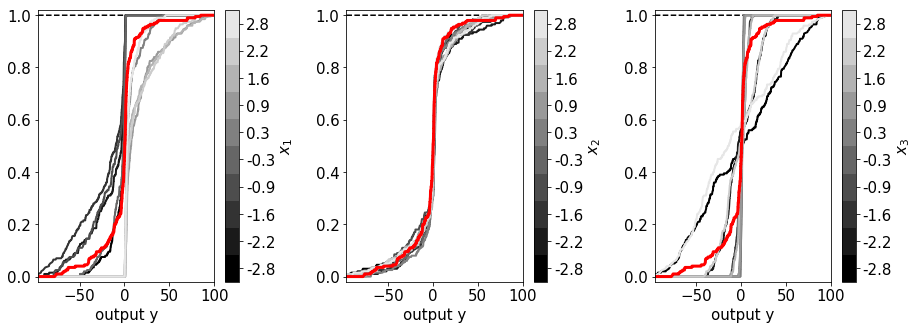Question:

From the CDF plots, which inputs factor would you say is influential? Why?

Are these results consistent with the visual analysis of the scatter plots?

The CDFs show that $x_{1}$ and $x_{3}$ are influential, as for both inputs their conditional and unconditional CDFs are different, which means that the effect on the output of fixing one input is different from the effect when all inputs are varying.

This is not true for $x_{2}$, where all CDFs are more or less overlapping, therefore it shows his influence is minimal.

### Step 7: Estimate the Kolmogorov-Smirnov statistics¶

The Kolmogorov-Smirnov (KS) statistic is the maximum absolute difference between the unconditional and the conditional CDFs:

$$\begin{equation}\ \text{KS}(\mathcal{I}_k) = \max_{y} | \hat{F}_y( y ) - \hat{F}_{y|x_i}( y | x_i \in \mathcal{I}_k ) | \end{equation}$$

$F_y( y )$ and $F_{y|x_i}( y | x_i )$ are the unconditional and conditional CDFs of the output $y$,

$\mathcal{I}_k$ are the $n$ intervals, with approximately the same number of values, into which each input factor $x_{i}$ has been split.

Since $F_{y|x_i}( y )$ accounts for what happens when the variability due to $x_i$ is removed, its distance from $F_y( y )$ provides a measure of the effect of $x_i$ on $y$.

The limit case is when $F_{y|x_i}( y )$ coincides with $F_y( y )$: it means that removing the uncertainty about $x_i$ does not affect the output distribution (i.e. $S_{x_{i}}$ = 0).

Instead if the distance between $F_{y|x_i}( y )$ and $F_y( y )$ increases, the influence of $x_i$ increases as well.

In :
# Compute and plot Kolmogorov-Smirnov (KS) statistics for each conditioning interval:
KS = PAWN.pawn_plot_ks(YF, FU, FC, xc, n_col=3, X_Labels=X_Labels)
plt.subplots_adjust(left=None, bottom=None, right=None, top=None, hspace=0, wspace=0.4)
plt.show()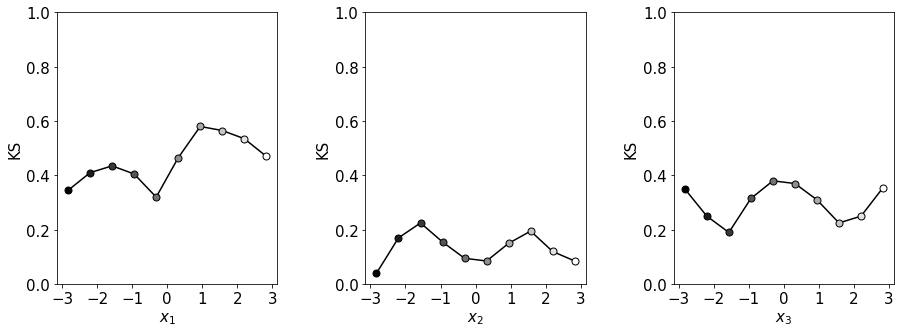Question:

Are the KS values consistent with the visual inspection of the CDF plots?

The KS values confirm that $x_{1}$ and $x_{3}$ are more influential than $x_{2}$, and also show that $x_{1}$ is more influential than $x_{3}$, which was difficult to see from the CDF plots.

### Step 8: Estimate the PAWN sensitivity indices¶

The PAWN sensitivity index is a statistic of the Kolmogorov-Smirnov statistics: $$\begin{equation}\ \hat{S}_i = \underset{k=1,...,n}{\text{stat}} \text{KS}(\mathcal{I}_k) \end{equation}$$

where

$stat$ is a statistic (e.g. median, maximum or mean).

In :
# Compute PAWN sensitivity indices:
KS_median, _, _ = PAWN.pawn_indices(X, Y, n)
# Plot results:
plt.rcParams['figure.figsize'] = [5, 5]
plt.figure()
pf.boxplot1(KS_median, X_Labels=X_Labels, Y_Label='KS (median)')
plt.show()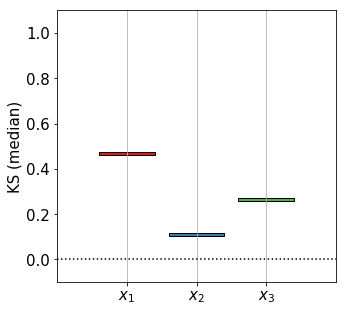Question:

Are these sensitivity indices values credible?

We can't really say. We need to assess the robustness of the results to the sample size used through bootstrapping.

### Step 9: Assess the robustness by bootstrapping¶

In order to assess the robustness of the estimated sensitivity indices, bootstrapping is performed (here we resample 1000 times).

The 95% confidence intervals of the sensitivity indices are plotted below.

In :
# Use bootstrapping to derive confidence bounds:
Nboot = 1000
# Compute sensitivity indices for Nboot bootstrap resamples
# (Warning: the following line may take some time to run, as the computation of
# CDFs is costly):
KS_median, _, _ = PAWN.pawn_indices(X, Y, n, Nboot=Nboot)
# KS_median has shape (Nboot, M)
# Compute mean and confidence intervals of the sensitivity indices across the
# bootstrap resamples:
KS_median_m, KS_median_lb, KS_median_ub = aggregate_boot(KS_median) # shape (M,)

# Plot bootstrapping results:
plt.figure()
pf.boxplot1(KS_median_m, S_lb=KS_median_lb, S_ub=KS_median_ub,
X_Labels=X_Labels, Y_Label='KS (median)')

plt.subplots_adjust(left=None, bottom=None, right=None, top=None, hspace=0, wspace=0.4)
plt.show()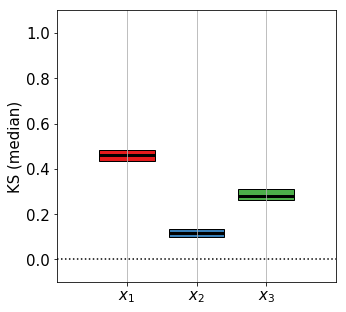Question:

Are the sensitivity indices adequately estimated? Is the sample size large enough?

Yes, as the confidence intervals of the sensitivity indices are not overlapping.

### Step 10: Screeening - Identify non influential inputs¶

The dummy parameter is a numerical artifice, with no influence on the model output, which is used to estimate the threshold for non-influential inputs.

Uninfluential input factors should have zero-valued sensitivity indices, but since sensitivity indices are computed by numerical approximations rather than analytical solutions, an uninfluential factor may still be associated with a non-zero (although small) index value.

Therefore if the index of an input factor is below the value of the sensitivity index of the dummy parameter, then the input factor is deemed uninfluential (Reference 8).

In :
# Identify influential and non-influential inputs adding an
# articial 'dummy' input to the list of the model inputs. The sensitivity
# indices for the dummy parameter estimate the approximation error of the
# sensitivity indices. For reference and more details, see help of the function
# PAWN.pawn_indices

# Name of parameters (will be used to customize plots) including the dummy input:
X_Labels_dummy=['x(1)', 'x(2)', 'x(3)', 'dummy']

# Sensitivity indices using bootstrapping for the model inputs and the dummy
# input:
# Use bootstrapping to derive confidence bounds:
Nboot = 1000
# Compute sensitivity indices for Nboot bootstrap resamples. We analyse KS_max
# only (and not KS_median and KS_mean) for screening purposes.
# (Warning: the following line may take some time to run, as the computation of
# CDFs is costly):
KS_median, _, _, KS_dummy = PAWN.pawn_indices(X, Y, n, Nboot=Nboot, dummy=True)
# KS_median has shape (Nboot, M), KS_dummy has shape (Nboot, )

# Compute mean and confidence intervals of the sensitivity indices across the
# bootstrap resamples:
KS_m, KS_lb, KS_ub = aggregate_boot(np.column_stack((KS_median, KS_dummy)))

# Plot bootstrapping results:
plt.figure() # plot main and total separately
pf.boxplot1(KS_m, S_lb=KS_lb, S_ub=KS_ub, X_Labels=X_Labels_dummy, Y_Label='KS')
plt.show()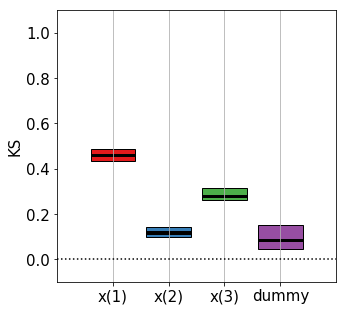Question:

Is $x_{2}$ non influential?

The confidence intervals of the sensitivity index of $x_{2}$ are overlapping with the ones of the dummy parameter, which means that $x_{2}$ is not influential, as its sensitivity index value is comparable to numerical approximation errors.
plt.rcParams['figure.figsize'] = [10, 10]